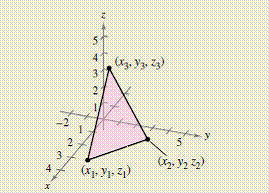Chapter 11.4, Problem 46E

Chapter
Section
Textbook Problem

# HOW DO YOU SEE IT? The vertices of a triangle in space are ( x 1 , y 1 , z 1 ) , ( x 2 , y 2 , z 2 ) , and ( x 3 , y 3 , y 3 ) . Explain how to find a vector perpendicular to the triangle.To determine

To Find:

A vector that is perpendicular to the triangle.

Explanation

Given: The vertices of a triangle in space are (x1,y1,z1),(x2,y2,z2) and (x3,y3,z3) Sketch is as shown below:

Calculation:

Vertices of a triangle are (x1,y1,z1),(x2,y2,z2) and (x3,y3,z3).

The diagram as shown.

If there are two non-zero vectors u and v, then cross product u×v is perpendicular to both vectors u and v.

So firstldetermine the component form of vectors AB and AC on the same plane.

AB=<x2,y2,z2><x1,y1,z1=<x2x1,y2y1,z2z1>

And,

### Still sussing out bartleby?

Check out a sample textbook solution.

See a sample solution

#### The Solution to Your Study Problems

Bartleby provides explanations to thousands of textbook problems written by our experts, many with advanced degrees!

Get Started